Courses

# Competition Level Test: States of Matter: Gases & Liquids

## 30 Questions MCQ Test Chemistry for JEE | Competition Level Test: States of Matter: Gases & Liquids

Description
This mock test of Competition Level Test: States of Matter: Gases & Liquids for JEE helps you for every JEE entrance exam. This contains 30 Multiple Choice Questions for JEE Competition Level Test: States of Matter: Gases & Liquids (mcq) to study with solutions a complete question bank. The solved questions answers in this Competition Level Test: States of Matter: Gases & Liquids quiz give you a good mix of easy questions and tough questions. JEE students definitely take this Competition Level Test: States of Matter: Gases & Liquids exercise for a better result in the exam. You can find other Competition Level Test: States of Matter: Gases & Liquids extra questions, long questions & short questions for JEE on EduRev as well by searching above.
QUESTION: 1

### If density of vapours of a substance of molar mass 18 gm / mole at 1 atm pressure and 500 K is 0.36 kg m-3 , then value of Z for the vapours is : (Take R = 0.082 L atm mole K-1)

Solution: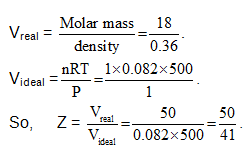QUESTION: 2

### Equal amount (mass) of methane and ethane have their total translational kinetic energy in the ratio            3: 1 then their temperatures are in the ratio.

Solution: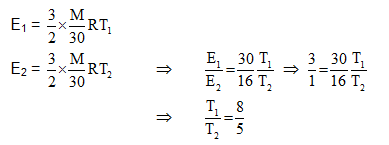QUESTION: 3

### Which of the following sketches is an isobar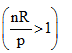Solution: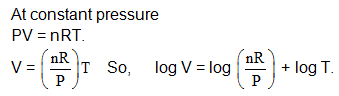QUESTION: 4

For a certain gas which deviates a little from ideal behaviour, the values of density, p were measured at different values of pressure, P. The plot of Pip on the Y-axis versus P on the X-axis was nonlinear and had an intercept on the Y-axis, which was equal

Where M = molar mass.

Solution:

For constant pressure V α T, hence linear dependence with the slope

QUESTION: 5

A sample of water gas has a composition by volume of 50% H2, 45% CO and 5% CO2. Calculate the volume in litre at S.T.P. of water gas which on treatment with excess of stream will produce 5 litre H2. The equation for the reaction is :        CO + H2O → CO2   + H

Solution: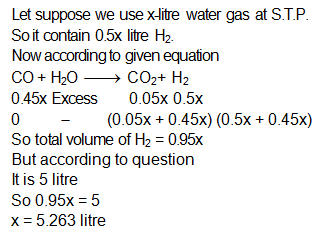QUESTION: 6

Flask A of volume 10 liter containing 20 gram of H2 and flask B of volume 10 litre containing 88 gram CO2 are connected by a connector having negligible volume. When valve of the connector is opened what is the composition of H2 gas in flask B after openening the valve.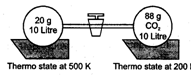Solution: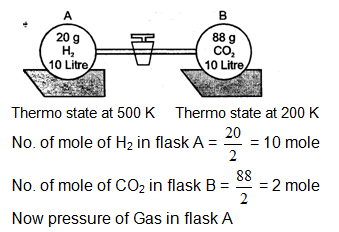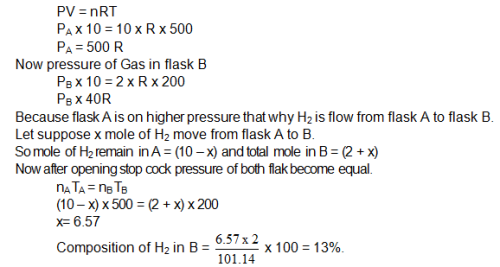QUESTION: 7

Consider the reaction, 2X(g) + 3Y(g) → Z(g)

Where gases X and Y are insoluble and inert to water and Z form a basic solution. In an experiment 3 mole each of X and Y are allowed to react in 15 lit flask at 500 K. When the reaction is complete, 5L of water is added to the flask and temperature is reduced to 300 K. The pressure in the flask is (neglect aqueous tension)

Solution:

The reaction is
2X(g) + 3Y(g) → Z(g)
2 moles of X reacts with 3 moles of Y to form 1mole of Z.
Now 3 moles of X and 3 moles of Y are present in the flask. Y is the limiting reagent. (Tocheck LR, divide the no of moles by stoichiometric coff. of that element. The least value will be LR)
After reaction, 1 mole of X is remaining and 1 mole of Z is formed, X is insoluble in water whereas Z is soluble in water.
Thus, the pressure in the flask is due to 1 mole of X.
The total volume of the flask is 15 L. The volume of water is 5 L. Thus the volume of gas is 15-5 = 10L.
The ideal gas equation is pV = nRT
Hence ,
p = nRT/V = 1×0.0821×300/10
= 2.46 atm

QUESTION: 8

Change from liquid in to gaseous state is known as

Solution:

Changing of liquid in a gaseous state is termed as evaporation.

QUESTION: 9

There are n containers having volumes V, 2V, ……, nV. A fixed mass of a gas is filled in all containers connected with stopcock. All containers are at the same temperature if pressure of first container is P then final pressure when all stopcocks are opened is:

Solution: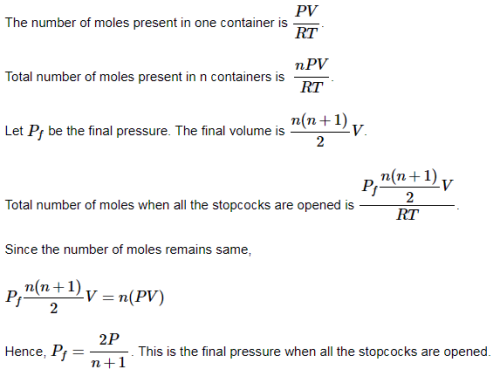QUESTION: 10

SO2 and CH4 are introduced in a vessel in the molar ratio 1 : 2. The ratio of molecules of two gases present in the container when their rate becomes equal is

Solution:

The ratio of the number of moles of SO2 to the number of moles of  CH4 is n1/n2= 1/2
​Here, 1 represents  SO2 and 2 represents CH4
The rate of effusion is proportional to the ratio of pressure to the square root of molecular weight.
r∝p/√M
The rates of effusions of two gases are equal.
p1/√M1 = p2/√M2
​The number of moles are proportional to pressure.
Hence,
p1/p2 = √(M1/M2) = n1/n2
​Thus, n1/n2= √(M1/M2)=√(64/16)=2:1

QUESTION: 11

A mixture of two gases A and B in the mole ratio 2 : 3 is kept in a 2 litre vessel. A second 3 litre vessel has the same two gases in the mole ratio 3 : 5. Both gas mixtures have the same temperature and same total pressure. They are allowed to intermix the final temperature and total pressure are the same as the initial values, the final volume being 5 litres. Given that the molar masses are MB and MB, what is the mean molar mass of the final mixture?

Solution: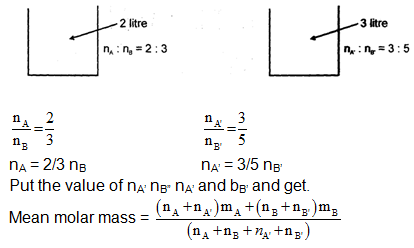QUESTION: 12

A 2 m long tube closed at one end is lowered vertically into' water until the closed end is flush with the water surface. See figure below. Calculate the water level height in the tube. (Barometric pressure -1 atm =10 m of hydrostatic water head, Temperature = 25°C, Density of, water = 1.00 g/ml. Neglect water vapour pressure).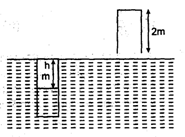Solution:

Applying Boyle’s law
P1V1 = P2V21
10Pw.2 = (10+h)pw
h = 1.7 m
Height of water level in the tube = 2-h = 2-1.71 = 0.29 m

QUESTION: 13

At a certain temperature for which RT = 25 lit. atm. mot -1 , the density of a gas, in gm lit-1, is d = 2.00 P + 0.020 P2, where P is the pressure in atmosphere. The molecular weight of the gas in gm mot -1 is

Solution: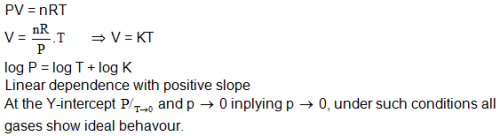QUESTION: 14

A mixture of carbon monoxide and carbon dioxide is found to have a density of 1.7 g/lit at S.T.P. The mole fraction of carbon monoxide is

Solution:

The average molar mass is M=ρRT/P=
(1.7g/L×0.0821Latm/mol/K×273K) / 1atm =38.1g/mol
Let x be the mole fraction of CO. The mole fraction of CO2 will be 1−x
The molar masses of CO and CO2 are 28 g/mol and 44 g/mol respectively.
The average molar mass of the mixture is, 38.1=28x+44(1−x)/1
​Hence, x=0.37
Hence, the mole fraction of CO is 0.37.

QUESTION: 15

If equal weights of oxygen and nitrogen are placed in separate containers of equal volume at the same temperature, which one of the following statements is true?

(mol wt: N2 = 28, O2 = 32)

Solution:

PV = nRT
P = nRT/V
P = (mass / Mmolecular wt) RT/V
at the same mass, R, T and V, higher Mmolecular wt → lower Pressure.
O2 is 32 g/mole and N2 is 28 g/mole.
So, O2 is lower pressure than N2

QUESTION: 16

The molecular radius for a certain gas = 1.25 A. What is a reasonable estimate of the magnitude of the van der Wails constant, b, for the gas?

Solution:

b=4×4/3πr3×NA
​b=16/3πr3×NA
​r=1.25×10−10m
b=16/3×22/7(1.25×10−10)3×6.022×1023m3
To convert in litre, 1m3=1000 or 103 litres
b=1.97×10−2 lit/mol
Hence, option C is correct

QUESTION: 17

Given the value of their van der Wails' constant 'a' arrange the following gases in the order of their expected liquification pressure at a temperature T. T is below the critical point of all the gases.            Gas                             CH4                             Kr                             N2           Cl2

‘a’ (atmL2. Mol-1)       2.283                          2.439                       1.408     6.579

Solution:

More the value of ‘a’, more is the attraction b/w molecules. And so less pressure will be needed to liquify the gas.
S, the order will be Cl2 < Kr < CH4 < N2

QUESTION: 18

The critical volume of a gas is 0.072 lit. mo1-1. The radius of the molecule will be, in cm

Solution:

The critical volume of three times the Van der Waals constant 'b'.
b=16/3πr3NA;Vc=3b=0.072 lit. mol−1
The expression for the magnitude of the Van der Waals constant, b, for the gas is b=16/3πr3NA
​Thus 3b=16/3πr3NA×3
Substitute values in the above expression,
0.072×1000=16/3×6.023×1023×π×r3×3
Thus r=(3/4π×10−23)1/3cm.

QUESTION: 19

The van der Waal's constant 'b' for N2 and H2 has the values 0.039 lit moil-1 and 0.0266 lit mo1-1. The density of solid N2 is 1 g cm-3. Assuming the molecules in the solids to be close packed with the same percentage void, the density of solid H2 would be (in g cm-3)

Solution:

The expression for the magnitude of the van der Waals constant, b, the volume V, molecular weight M and density d for nitrogen and hydrogen is as given below: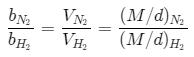Substitute values in the above expression: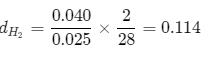Thus the density of hydrogen is 0.114 gcm−3.

QUESTION: 20

Which of the following statements is not true?

Solution:

The square of the mean speed of the molecules is not equal to the mean squared speed at a certain temperature.
The square of the mean speed of the molecules is (C1+C2+C3+....Cn)2/n
The mean squared speed is = C12+C22+C32+....Cn2 / n

QUESTION: 21

If the number of molecules of SO2 (molecular weight = 64) effusing through an orifice of unit area of cross-section in unit time at 0°C and 1 atm pressure is n. The number of He molecules (atomic weight = 4) effusing under similar conditions at 273°C and 0.25 atm is

Solution:

The number of molecules of SO2 (molecular weight =64) effusing through an orifice of unit area of the cross-section in unit time at 0°C and 1 atm pressure is n. This represents the rate of effusion of SO2.
Let x be the rate of effusion of He.
The expression for the relative rates of effusion of two gases is
r1/r2 = p1/p2√(T2M2/T1M1)
​Here, 1 represents SO 2 and 2 represents He.
Substitute values in the above expression.
n/x=1/0.25 √[(273×64)/(2×273×4)]
​Hence,  x= n/√2
​So, the correct option is A

QUESTION: 22

A capillary tube of uniform diameter contains gas samples A and B, separated by a short column of Hg, L mm in length. The ends are sealed. In horizontal position, the confined gases occupy 'a' mm and 'b' mm in length with a common unknown pressure (P). In vertical position, the lengths become respectively a' mm and b' mm. Then P is equal to

Solution:

By ideal gas law: PV=nRT
For a particular gas since n and T are constant.  ⇒PV=Constant
⇒  For gas A
Pa=PAa′..............(i) (Here length (a) is directly proportional to volume. Hence it can be used in place of volume.)
⇒ For gas B
Pb=PBb′.......(ii)
Since gas B is supporting l length of mercury over it in addition to gas A
⇒PB=PA+ρgl..........(iii) ( ρ is the density of mercury)
Putting (iii) in (ii)
⇒Pb=(PA+ρgl)b′............(iv)
Eliminating  PA
from (i) and (iv)
⇒Pb=Pa/a′b′+ρglb′⇒Pba′−ab′)/a’=ρglb′
⇒P=ρgla′b′/ba’-ab’(in SI units)
⇒P in mm of Hg =dP(in SI)/ρg
P(mmHg)=la′b′/ba′-ab′ = l/(b/b′) - (a/a′)
Thus (a) is the correct option

QUESTION: 23

Two glass bulb A and B are connected by a very small tube (of negligible volume) having stop cock. Bulb A has a volume of 100 cm3 and contains certain gas while bulb B is empty. On opening the stop cock, the pressure in 'A' fell down by 60%. The volume of bulb B must be

Solution:

Let the initial pressure in bulb A be P.
Given that the pressure fell down 40% on opening the stop cock
∴ Final pressure = 40/100P = 2/5P
Initial volume of bulb A =100cm3
Let the initial volume of bulb B be V.
∴ total volume after opening the stopcock =100+V
By using Boyle's law,
PiVi=PfVf
P×100=2/5P×(100+V)
⇒V=150cm3
Hence, the volume of bulb B must be 150cm3 or 150 mL(1 cm3 = 1 mL)

QUESTION: 24

A certain gas effuses out of two different vessels A and B. A has a circular orifice while B has a square orifice of length equal to the radius of the orifice of vessel A. The ratio of rate of diffusion of the gas from vessel A to that from vessel B is

Solution:

More the area more the effusion.
∴r1/r2 = A1/A2
r1/r2 = πr2/r2 = π

QUESTION: 25

If 1023 gas molecules each having a mass of 1a-25 kg, placed in a 1 L container, moving with rms speed of 105 cm/sec. then the total KE of gasseous molecules and pressure exerted by molecules, respectively are:

Solution: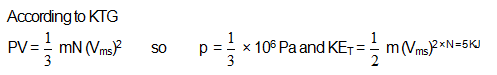QUESTION: 26

Statement-1: The value of vender wail's constant (a) is larger for ammonia than for nitrogen.

Statement-2: Hydrogen bonding is present in ammonia.

Solution:

Due to presence of H-bonding in NH3, the value of Van der waals constant (a) is larger for ammonia than for nitrogen. As ‘a’ is the measure of attractive forces, H-bonding increases its value.

QUESTION: 27

Statement-1: At constant temperature of pressure on the gas is doubled, Density is also doubled.

Statement-2: At constant temperature molecular mass of a gas is directly proportional to the density and inversely proportional to the pressure.

Solution:

We have pV = nRT, pV = wt/Mol wt RT = pM = dRT
Or p∝d. So increase in pressure will be matched by the same amount of increases in density.Molecular mass does not depend upon T, P.
So option c is correct.It should be Statement-1 is True, Statement-2 is False.

QUESTION: 28

Statement-1: At Boyle's temperature a real gas behaves as ideal gas for wide range of temperature and pressure.

Statement-2: At Boyle's temperature vanderwaal constant 'a' and b compensate each other.

Solution:

At Boyle's temperature a real gas behaves as an ideal gas for a wide range of temperature and pressure.
This is because van der waals constant 'a' and 'b' compensate for each other at Boyle's temperature. At this temperature, the value of the compressibility factor 'z' is 1.

QUESTION: 29

Statement-1: The area under the maxwells distribution molecular speed curve remains same irrespective of temperature of gas.

Statement-2: The fraction of molecules with most probable speed increases with increase of temperature

Solution: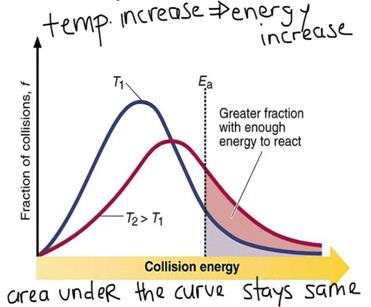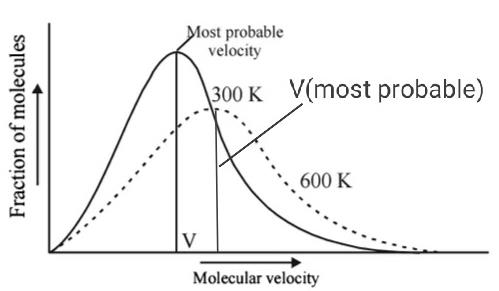So with increase in temperature, fraction of molecules with most probable velocity decreases.
Therefore, option d is correct.

QUESTION: 30

Statement-1: Greater is critical temperature more easily a gas can be liquefied.

Statement-2: Critical temperature depends on the magnitude of intermolecular forces of attraction between the gas.

Solution:

Yes, both statements are true and II is the correct explanation of I. The critical temperature of a gas is the temperature at or above which no amount of pressure, however great, will cause the gas to liquefy.
The reason for the change in critical temperature is the attractive forces acting b/w molecules.